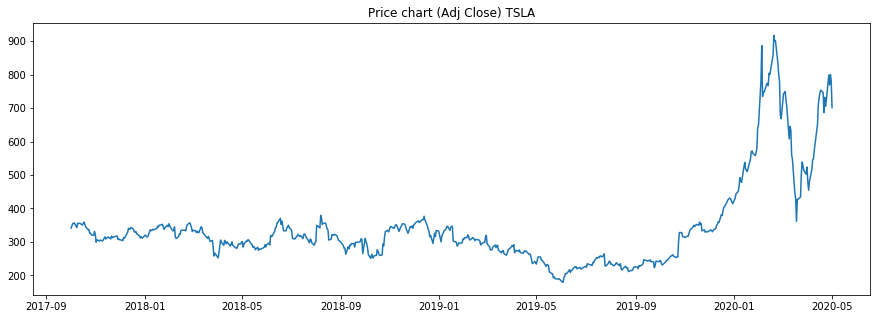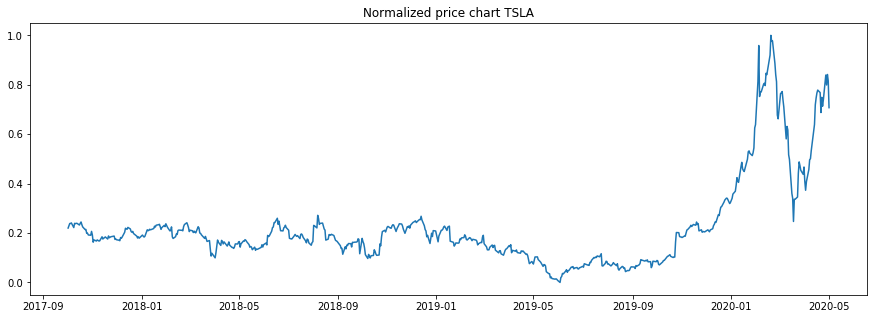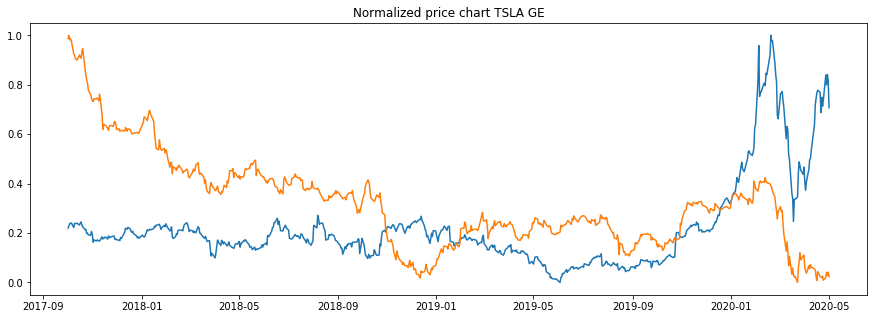In this article we will have a look at a way how to normalize time series data. Specifically we will be normalizing stock price data on daily timeframe. Normalizing time series data is benefitial when we want to compare multiple time series/stock price trends.

The benefit is that whatever the price range was originally, after normalization the price will be ranging in an interval between 0 and 1. So normalization is convenient for comparing price trends for multiple stocks in one graph.

Normalizing time series is also good as preprocessing technique for later usage in predictions via neural networks. Neural networks prefer standardized/normalized input so they can learn faster.

How to normalize time series:

    # time series normalization equation
y = (x - min) / (max - min)

x ... price from input time series
y ... normalized time series value
min ... minimum price in the time seies
max ... maximum price of the time series

#### Import libraries:¶

In :
#optional installations:

from IPython.core.display import display, HTML
display(HTML("<style>.container { width:100% !important; }</style>"))

# ___library_import_statements___
import pandas as pd

# for pandas_datareader, otherwise it might have issues, sometimes there is some version mismatch
pd.core.common.is_list_like = pd.api.types.is_list_like

# make pandas to print dataframes nicely
pd.set_option('expand_frame_repr', False)

import numpy as np
import matplotlib.pyplot as plt
import datetime
import time

import yfinance as yahoo_finance

#optional
#yahoo_finance.pdr_override()
%matplotlib inline


#### Select stock ticker and time window¶

In :
# ___variables___
ticker = 'TSLA'

start_time = datetime.datetime(2017, 10, 1)
#end_time = datetime.datetime(2019, 1, 20)
end_time = datetime.datetime.now().date().isoformat()         # today

In :
def get_data(ticker, start_time, end_time):

# yahoo gives only daily historical data
connected = False
while not connected:
try:
ticker_df = web.get_data_yahoo(ticker, start=start_time, end=end_time)
connected = True
print('connected to yahoo')
except Exception as e:
print("type error: " + str(e))
time.sleep( 5 )
pass

# use numerical integer index instead of date
ticker_df = ticker_df.reset_index()
return ticker_df

In :
df = get_data(ticker, start_time, end_time)

connected to yahoo
Date        High         Low        Open       Close    Volume   Adj Close
0 2017-10-02  343.700012  335.510010  342.519989  341.529999   5286800  341.529999
1 2017-10-03  348.549988  331.279999  335.899994  348.140015  10153600  348.140015
2 2017-10-04  358.619995  349.600006  351.250000  355.010010   8163500  355.010010
3 2017-10-05  357.440002  351.350006  356.000000  355.329987   4171700  355.329987
4 2017-10-06  360.100006  352.250000  353.100006  356.880005   4297500  356.880005


#### Define normalization function¶

In :
def normalize_data(df):
# df on input should contain only one column with the price data (plus dataframe index)
min = df.min()
max = df.max()
x = df

# time series normalization part
# y will be a column in a dataframe
y = (x - min) / (max - min)

return y

In :
df['norm'] = normalize_data(df['Adj Close'])

In :
print(df.head())
print(df.tail())

        Date        High         Low        Open       Close    Volume   Adj Close      norm
0 2017-10-02  343.700012  335.510010  342.519989  341.529999   5286800  341.529999  0.220137
1 2017-10-03  348.549988  331.279999  335.899994  348.140015  10153600  348.140015  0.229088
2 2017-10-04  358.619995  349.600006  351.250000  355.010010   8163500  355.010010  0.238391
3 2017-10-05  357.440002  351.350006  356.000000  355.329987   4171700  355.329987  0.238825
4 2017-10-06  360.100006  352.250000  353.100006  356.880005   4297500  356.880005  0.240924
Date        High         Low        Open       Close    Volume   Adj Close      norm
645 2020-04-27  799.489990  735.000000  737.609985  798.750000  20681400  798.750000  0.839299
646 2020-04-28  805.000000  756.690002  795.640015  769.119995  15222000  769.119995  0.799174
647 2020-04-29  803.200012  783.159973  790.169983  800.510010  16216000  800.510010  0.841682
648 2020-04-30  869.820007  763.500000  855.190002  781.880005  28471900  781.880005  0.816453
649 2020-05-01  772.770020  683.039978  755.000000  701.320007  32479600  701.320007  0.707360


### Plotting¶

Plot regular price chart:

In :
# plot price
plt.figure(figsize=(15,5))
plt.title('Price chart (Adj Close) ' + ticker)
plt.show()#### Plot normalized time series¶

When comparing to above picture we see that the trend looks identical with normalized time series as with the regular prices. The only difference is that normalized price time series ranges between 0 and 1. This makes it very convenient to plot multiple stocks in one picture to compare trends.

In :
# plot normalized price chart
plt.figure(figsize=(15,5))
plt.title('Normalized price chart ' + ticker)
plt.plot(df['Date'], df['norm'])

plt.show()#### Compare multiple stocks in normalized graph¶

Let's download data for another company and visualize both stocks in graph with normalized prices.

In :
ticker1='GE'
df1 = get_data(ticker1, start_time, end_time)

connected to yahoo
Date       High        Low       Open      Close      Volume  Adj Close
0 2017-10-02  23.663462  23.173077  23.288462  23.625000  44201200.0  22.511696
1 2017-10-03  23.875000  23.394230  23.663462  23.846153  35263800.0  22.722427
2 2017-10-04  23.932692  23.490385  23.923077  23.538462  33435300.0  22.429232
3 2017-10-05  23.625000  23.221153  23.451923  23.596153  36149200.0  22.484207
4 2017-10-06  23.596153  23.201923  23.471153  23.451923  42358900.0  22.346773

In :
# plot normalized price chart
plt.figure(figsize=(15,5))
plt.title('Normalized price chart ' + ticker + ' ' + ticker1)
plt.plot(df['Date'], df['norm'])
plt.plot(df1['Date'], df1['norm'])

plt.show()As we can see, now we can compare the trends in stock prices easily in the normalized graph.